# Photonic quantum neural networks

By Nathan Killoran, Josh Izaac, Juan Miguel Arrazola, and Thomas R. Bromley

At Xanadu we are developing a photonic quantum computer: a device that processes information stored in quantum states of light. We are very excited by the possibilities that this approach brings. Photonic quantum computers naturally use continuous degrees of freedom — like the amplitude and phase of light — to encode information. This continuous, or analog, structure makes photonic devices a natural platform for quantum versions of neural networks.

How do we mimic a neural network using a photonic system? And where does quantum enter the game?

This summer, we released an exciting new paper which resolves these questions. We propose a photonic circuit which consists of a sequence of repeating building blocks, or layers. Layers can be composed, with the output of one layer serving as the input to the next. These photonic layers are akin to the layers which appear in classical neural networks.

Classical nets take an input x, multiply it by a weight matrix W, add a bias b, and pass the result through a nonlinear function (such as tanh or ReLU):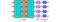The basic layer unit of a classical neural network, which performs the transformation x→Φ(Wx+b). Using the singular-value decomposition, W can equivalently be given by two orthogonal matrices O1, O2, and a diagonal matrix Σ.

Our quantum layer mimics this functionality using photonic quantum gates: interferometers (made from phase shifters and beamsplitters), squeezing and displacement gates, and a fixed nonlinear transformation. These are the same gates that are used to build a photonic quantum computer, so our quantum neural network architecture has all the power of quantum computers.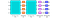The basic layer unit of a photonic quantum neural network. Gates are coloured to indicate which classical component they are related to.

The quantum neural network retains strong ties to classical neural networks. In fact, the quantum version can be used to run the classical version, by using the quantum net in a way which does not generate any quantum weirdness (superposition, entanglement, etc.). The similarity is illustrated through the colouring of gates in the two images above. The interferometers and squeezing gates are connected to the weight matrix, the displacement gates with the bias, and the quantum nonlinearity with the classical nonlinearity.

We trained the quantum neural network to do several tasks: curve fitting, fraud detection, a classical-quantum autoencoder, and generating images. We will highlight some of these cool applications in a future blog post. In the meantime, we’d like to share one particular and powerful use of photonic quantum neural networks.

# Learning quantum states and quantum gates

While it is easy to simulate arbitrary quantum states of light using the Strawberry Fields software package, sometimes we forget how much of a challenge generating them can be for quantum computing researchers — who might spend days rearranging an equation to only end up back where they started. As reported in another recent research paper, the quantum neural network architecture we have pioneered can help in these cases. Using training methods from machine learning, we can optimize a quantum neural network circuit to produce arbitrary quantum states. Once we have learned the correct parameters, this state-preparation subroutine can then be reused within other quantum circuits or algorithms.

A quantum computing expert might takes weeks or months to craft such a circuit, while the machine learning approach can find solutions on a timescale of hours. This can dramatically accelerate the research and development process, potentially leading to breakthroughs in quantum computing.

For example, one tricky problem in quantum experiments is the creation of single-photon states — currently done via a random process known as spontaneous parametric down-conversion. We trained a quantum neural network which can produce on-demand single photon states using a fixed set of quantum gates. Quantum states of light can be represented by smooth landscapes called Wigner functions, so we can visualize the state output by our quantum neural network during training with a 3D animation: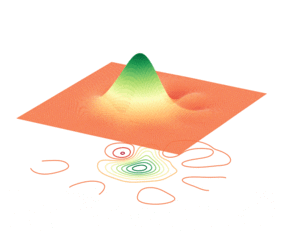The output of the quantum neural network at different stages of training. In these plots, we always begin from a fixed starting state (shown by the initial peak, called a Gaussian). We then gradually learn to output a single photon state (shown here by the final shape with a red trough at the center).

We can even do this with more complicated states, such as the so-called Schrödinger cat states, or the ON state — an important state in photonic quantum computing, used to construct various quantum gates.

Going one step further, our paper shows how to implement quantum gates using the quantum neural network architecture. Gates are another important ingredient in quantum computation, giving us a tool to control how quantum systems evolve. All quantum algorithms, including the famous Shor’s algorithm, require gates to function, yet it can be hard to work out how to implement them physically. Our approach automates this procedure.

To visualize this process, we can use the fact that gates in quantum computing are unitary matrices, with complex-valued entries. We can thus depict a gate graphically by colouring the real and imaginary entries of the corresponding matrix according to their magnitude.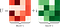A random four-dimensional gate U, depicted using the real and imaginary parts of the corresponding unitary matrix.

The transformation performed by our quantum neural network archicture can also be represented with this simple colouring scheme. The image below demonstrates how the quantum neural network transformation — initially random — is progressively trained to match a specific target gate.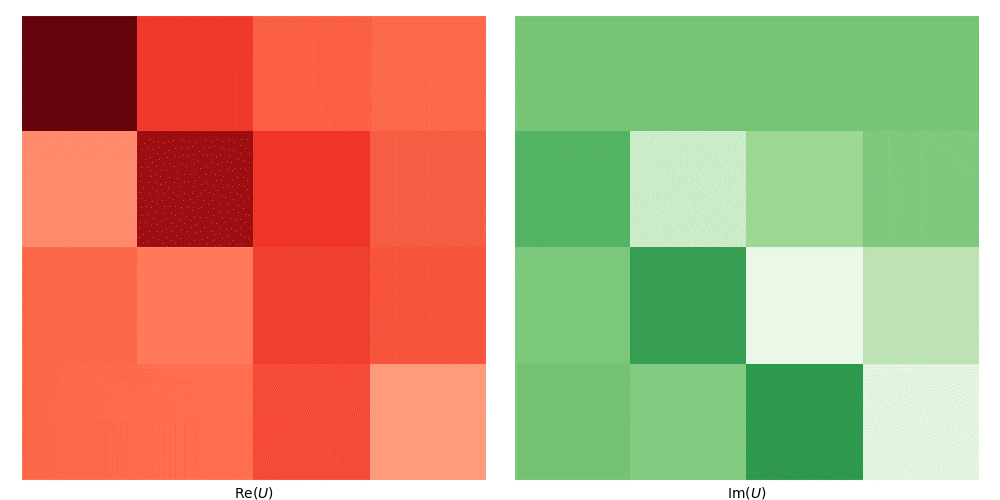Each frame in the above animation corresponds to the transformation carried out by a quantum neural network at a particular step in training. At the end of training, the transformation closely matches the desired one given above by U.

All the code for our state and gate learning is available on GitHub, and uses Python, the machine learning framework TensorFlow, and our quantum simulation software Strawberry Fields. Download it and see what states you can generate!

Written by

Written by

#### Building quantum computers that are useful and available to people everywhere.